# How to drop one or multiple columns in Pandas Dataframe

• Difficulty Level : Basic
• Last Updated : 05 Jul, 2022

Let’s discuss how to drop one or multiple columns in Pandas Dataframe. To Delete a column from a Pandas DataFrame or Drop one or more than one column from a DataFrame can be achieved in multiple ways.

Create a simple Dataframe with dictionary of lists, say column names are A, B, C, D, E. In this article, we will cover 6 different methods to delete some columns from Pandas DataFrame.

## Python3

 `# Import pandas package ` `import` `pandas as pd ` ` `  `# create a dictionary with five fields each ` `data ``=` `{ ` `    ``'A'``: [``'A1'``, ``'A2'``, ``'A3'``, ``'A4'``, ``'A5'``], ` `    ``'B'``: [``'B1'``, ``'B2'``, ``'B3'``, ``'B4'``, ``'B5'``], ` `    ``'C'``: [``'C1'``, ``'C2'``, ``'C3'``, ``'C4'``, ``'C5'``], ` `    ``'D'``: [``'D1'``, ``'D2'``, ``'D3'``, ``'D4'``, ``'D5'``], ` `    ``'E'``: [``'E1'``, ``'E2'``, ``'E3'``, ``'E4'``, ``'E5'``]} ` ` `  `# Convert the dictionary into DataFrame ` `df ``=` `pd.DataFrame(data) ` ` `  `df `

Output: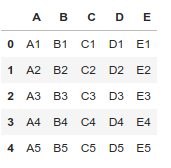### Method 1: Drop Columns from a Dataframe using drop() method.

Example 1: Remove specific single columns.

## Python3

 `# Import pandas package ` `import` `pandas as pd ` ` `  `# create a dictionary with five fields each ` `data ``=` `{ ` `    ``'A'``: [``'A1'``, ``'A2'``, ``'A3'``, ``'A4'``, ``'A5'``], ` `    ``'B'``: [``'B1'``, ``'B2'``, ``'B3'``, ``'B4'``, ``'B5'``], ` `    ``'C'``: [``'C1'``, ``'C2'``, ``'C3'``, ``'C4'``, ``'C5'``], ` `    ``'D'``: [``'D1'``, ``'D2'``, ``'D3'``, ``'D4'``, ``'D5'``], ` `    ``'E'``: [``'E1'``, ``'E2'``, ``'E3'``, ``'E4'``, ``'E5'``]} ` ` `  `# Convert the dictionary into DataFrame ` `df ``=` `pd.DataFrame(data) ` ` `  `# Remove column name 'A' ` `df.drop([``'A'``], axis``=``1``) `

Output: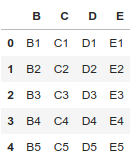Example 2: Remove specific multiple columns.

## Python3

 `# Import pandas package ` `import` `pandas as pd ` ` `  `# create a dictionary with five fields each ` `data ``=` `{ ` `    ``'A'``: [``'A1'``, ``'A2'``, ``'A3'``, ``'A4'``, ``'A5'``], ` `    ``'B'``: [``'B1'``, ``'B2'``, ``'B3'``, ``'B4'``, ``'B5'``], ` `    ``'C'``: [``'C1'``, ``'C2'``, ``'C3'``, ``'C4'``, ``'C5'``], ` `    ``'D'``: [``'D1'``, ``'D2'``, ``'D3'``, ``'D4'``, ``'D5'``], ` `    ``'E'``: [``'E1'``, ``'E2'``, ``'E3'``, ``'E4'``, ``'E5'``]} ` ` `  `# Convert the dictionary into DataFrame ` `df ``=` `pd.DataFrame(data) ` ` `  `# Remove two columns name is 'C' and 'D' ` `df.drop([``'C'``, ``'D'``], axis``=``1``) ` ` `  `# df.drop(columns =['C', 'D']) `

Output: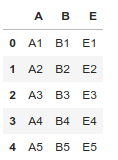Example 3: Remove columns based on column index.

## Python3

 `# Import pandas package ` `import` `pandas as pd ` ` `  `# create a dictionary with five fields each ` `data ``=` `{ ` `    ``'A'``: [``'A1'``, ``'A2'``, ``'A3'``, ``'A4'``, ``'A5'``], ` `    ``'B'``: [``'B1'``, ``'B2'``, ``'B3'``, ``'B4'``, ``'B5'``], ` `    ``'C'``: [``'C1'``, ``'C2'``, ``'C3'``, ``'C4'``, ``'C5'``], ` `    ``'D'``: [``'D1'``, ``'D2'``, ``'D3'``, ``'D4'``, ``'D5'``], ` `    ``'E'``: [``'E1'``, ``'E2'``, ``'E3'``, ``'E4'``, ``'E5'``]} ` ` `  `# Convert the dictionary into DataFrame ` `df ``=` `pd.DataFrame(data) ` ` `  `# Remove three columns as index base ` `df.drop(df.columns[[``0``, ``4``, ``2``]], axis``=``1``, inplace``=``True``) ` ` `  `df `

Output: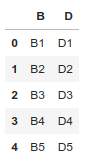### Method 2: Drop Columns from a Dataframe using iloc[] and drop() method.

Remove all columns between a specific column to another column.

## Python3

 `# Import pandas package ` `import` `pandas as pd ` `# create a dictionary with five fields each ` `data ``=` `{ ` `    ``'A'``: [``'A1'``, ``'A2'``, ``'A3'``, ``'A4'``, ``'A5'``], ` `    ``'B'``: [``'B1'``, ``'B2'``, ``'B3'``, ``'B4'``, ``'B5'``], ` `    ``'C'``: [``'C1'``, ``'C2'``, ``'C3'``, ``'C4'``, ``'C5'``], ` `    ``'D'``: [``'D1'``, ``'D2'``, ``'D3'``, ``'D4'``, ``'D5'``], ` `    ``'E'``: [``'E1'``, ``'E2'``, ``'E3'``, ``'E4'``, ``'E5'``]} ` ` `  `# Convert the dictionary into DataFrame ` `df ``=` `pd.DataFrame(data) ` ` `  `# Remove all columns between column index 1 to 3 ` `df.drop(df.iloc[:, ``1``:``3``], inplace``=``True``, axis``=``1``) ` ` `  `df `

Output: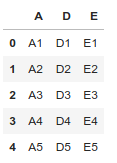### Method 3: Drop Columns from a Dataframe using ix() and drop() method.

Remove all columns between a specific column name to another column’s name.

## Python3

 `# Import pandas package ` `import` `pandas as pd ` ` `  `# create a dictionary with five fields each ` `data ``=` `{ ` `    ``'A'``: [``'A1'``, ``'A2'``, ``'A3'``, ``'A4'``, ``'A5'``], ` `    ``'B'``: [``'B1'``, ``'B2'``, ``'B3'``, ``'B4'``, ``'B5'``], ` `    ``'C'``: [``'C1'``, ``'C2'``, ``'C3'``, ``'C4'``, ``'C5'``], ` `    ``'D'``: [``'D1'``, ``'D2'``, ``'D3'``, ``'D4'``, ``'D5'``], ` `    ``'E'``: [``'E1'``, ``'E2'``, ``'E3'``, ``'E4'``, ``'E5'``]} ` ` `  `# Convert the dictionary into DataFrame ` `df ``=` `pd.DataFrame(data) ` ` `  `# Remove all columns between column name 'B' to 'D' ` `df.drop(df.ix[:, ``'B'``:``'D'``].columns, axis``=``1``) `

Output: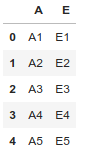### Method 4: Drop Columns from a Dataframe using loc[] and drop() method.

Remove all columns between a specific column name to another column’s name.

## Python3

 `# Import pandas package ` `import` `pandas as pd ` ` `  `# create a dictionary with five fields each ` `data ``=` `{ ` `    ``'A'``: [``'A1'``, ``'A2'``, ``'A3'``, ``'A4'``, ``'A5'``], ` `    ``'B'``: [``'B1'``, ``'B2'``, ``'B3'``, ``'B4'``, ``'B5'``], ` `    ``'C'``: [``'C1'``, ``'C2'``, ``'C3'``, ``'C4'``, ``'C5'``], ` `    ``'D'``: [``'D1'``, ``'D2'``, ``'D3'``, ``'D4'``, ``'D5'``], ` `    ``'E'``: [``'E1'``, ``'E2'``, ``'E3'``, ``'E4'``, ``'E5'``]} ` ` `  `# Convert the dictionary into DataFrame ` `df ``=` `pd.DataFrame(data) ` ` `  `# Remove all columns between column name 'B' to 'D' ` `df.drop(df.loc[:, ``'B'``:``'D'``].columns, axis``=``1``) `

Output: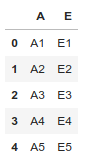Note: Different loc() and iloc() is iloc() exclude last column range element.

### Method 5: Drop Columns from a Dataframe in an iterative way.

Remove all columns between a specific column name to another column’s name.

## Python3

 `# Import pandas package ` `import` `pandas as pd ` ` `  `# create a dictionary with five fields each ` `data ``=` `{ ` `    ``'A'``: [``'A1'``, ``'A2'``, ``'A3'``, ``'A4'``, ``'A5'``], ` `    ``'B'``: [``'B1'``, ``'B2'``, ``'B3'``, ``'B4'``, ``'B5'``], ` `    ``'C'``: [``'C1'``, ``'C2'``, ``'C3'``, ``'C4'``, ``'C5'``], ` `    ``'D'``: [``'D1'``, ``'D2'``, ``'D3'``, ``'D4'``, ``'D5'``], ` `    ``'E'``: [``'E1'``, ``'E2'``, ``'E3'``, ``'E4'``, ``'E5'``]} ` ` `  `# Convert the dictionary into DataFrame ` `df ``=` `pd.DataFrame(data) ` `for` `col ``in` `df.columns: ` `    ``if` `'A'` `in` `col: ` `        ``del` `df[col] ` ` `  `df `

Output: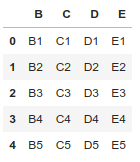## Python3

 `# Import pandas package ` `import` `pandas as pd ` ` `  `# create a dictionary with five fields each ` `data ``=` `{ ` `    ``'A'``: [``'A1'``, ``'A2'``, ``'A3'``, ``'A4'``, ``'A5'``], ` `    ``'B'``: [``'B1'``, ``'B2'``, ``'B3'``, ``'B4'``, ``'B5'``], ` `    ``'C'``: [``'C1'``, ``'C2'``, ``'C3'``, ``'C4'``, ``'C5'``], ` `    ``'D'``: [``'D1'``, ``'D2'``, ``'D3'``, ``'D4'``, ``'D5'``], ` `    ``'E'``: [``'E1'``, ``'E2'``, ``'E3'``, ``'E4'``, ``'E5'``]} ` ` `  `# Convert the dictionary into DataFrame ` `df ``=` `pd.DataFrame(data) ` `df.pop(``'B'``) ` ` `  `df `

Output:

```A    C    D    E
0    A1    C1    D1    E1
1    A2    C2    D2    E2
2    A3    C3    D3    E3
3    A4    C4    D4    E4
4    A5    C5    D5    E5```

My Personal Notes arrow_drop_up
Recommended Articles
Page :